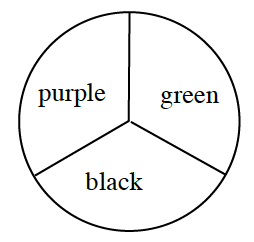### Home > CC2 > Chapter Ch5 > Lesson 5.3.2 > Problem5-110

5-110.The spinner at right is spun twice. Make a table and calculate the probability of each of the following outcomes.

1. Getting black twice.

What is the probability of getting black once? Multiply that by itself to find the probability of getting black twice.

$\left(\frac{1}{3}\right)\left(\frac{1}{3}\right)=\frac{1}{9}$

2. Getting green twice.

Is it any more or less likely that someone will get green over black?